Home Return

# My Report about PIC16F84A Microcontroller Implementation on Verilog Hardware Description Language

source codeView My Stats

word number: 9946

Time: 2021-04-21 07:57:08 +0000

## Note

This is one of my Doctoral assignment from Advanced Computer Architecture II Course which has never been published anywhere and I, as the author and copyright holder, license this assignment customized CC-BY-SA where anyone can share, copy, republish, and sell on condition to state my name as the author and notify that the original and open version available here.

## 1. Introduction

Peripheral interface controller (PIC) is a family of microcontrollers made by Microchip Technology. A microcontroller is a one chip computer that include microprocessors, memories, and peripherals. PIC devices are popular with both industrial developers and hobbyists due to their low cost, wide availability, large user base, extensive collection of application notes, availability of low cost or free development tools, serial programming, and re-programmable Flash-memory capability. They can be programmed to be timers, to control a production line, to control light and sound intensity by involving few sensors, and to perform other kind of tasks. The PIC microcontroller have five basic instruction cycle which are fetch, decode, execute, memory, and write (FDEMW) . 

## 2. Verilog HDL Design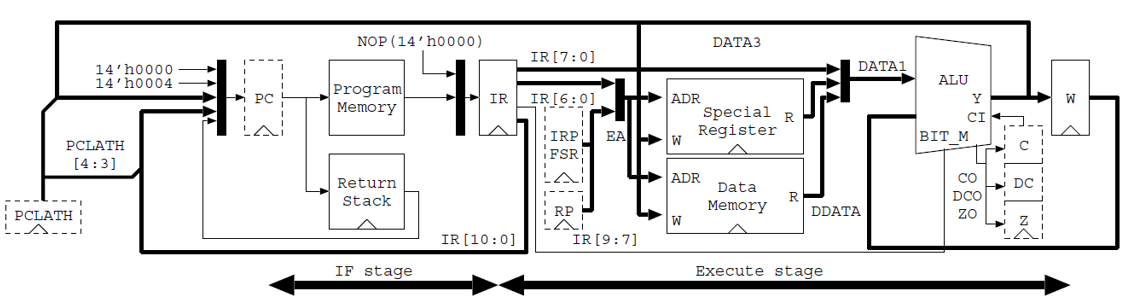Figure 1. This report’s design 

On the verilog hardware description language (HDL) design is based on Figure 1. This sections starts by constructing the arithmetic logic unit (ALU), bitmask, and W register. Then continue to design the program counter and return stack which its values to be sent to the instruction register where there is also decode and control behavior. Next is the design of special register, although the effective addressing is discussed in early part. After that the built module have to be connected to the firstly created ALU, bitmask, and W register. Lastly implement sleep and tristate buffer.

### 2.1 Arithmetic Logic Unit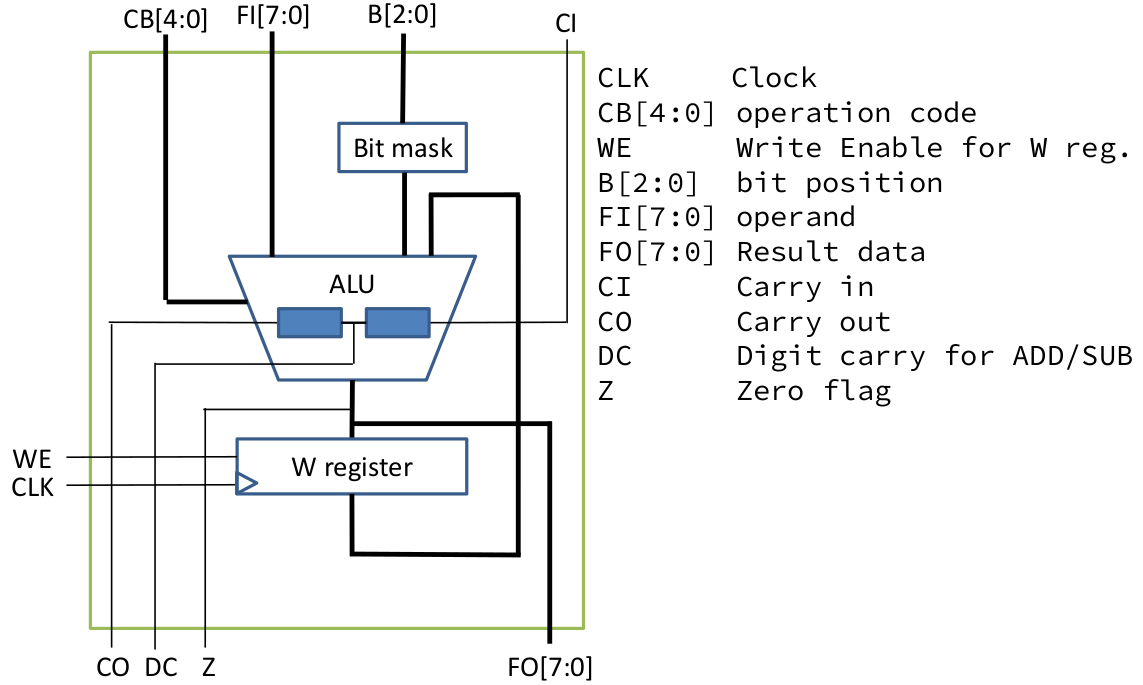Figure 2. ALU diagram 

Code 1. Input and output of ALU

```module alu ( CLK, CB, WE, B, FI, FO, CI, CO, DC, Z );
input CLK;// Clock
input [4:0] CB; // operation code
input WE; // Write enable for W register
input [2:0] B; // bit position
input [7:0] FI; // operand
input CI; // Carry in
output [7:0] FO; // dest bus
output CO; // Carry out
output DC; // Half carry
output Z; // Zero
reg HC; // half carry
reg [7:0] W;
reg [8:0] tmp;
reg [7:0] bit_mask;
wire sub;
assign sub = ( CB == `ISUB );
```

Code 2.\ Bitmask

```always @( B )
case( B )
3'b000: bit_mask = 8'b0000_0001;
3'b001: bit_mask = 8'b0000_0010;
3'b010: bit_mask = 8'b0000_0100;
3'b011: bit_mask = 8'b0000_1000;
3'b100: bit_mask = 8'b0001_0000;
3'b101: bit_mask = 8'b0010_0000;
3'b110: bit_mask = 8'b0100_0000;
3'b111: bit_mask = 8'b1000_0000;
default: bit_mask = 8'bxxxx_xxxx;
endcase
```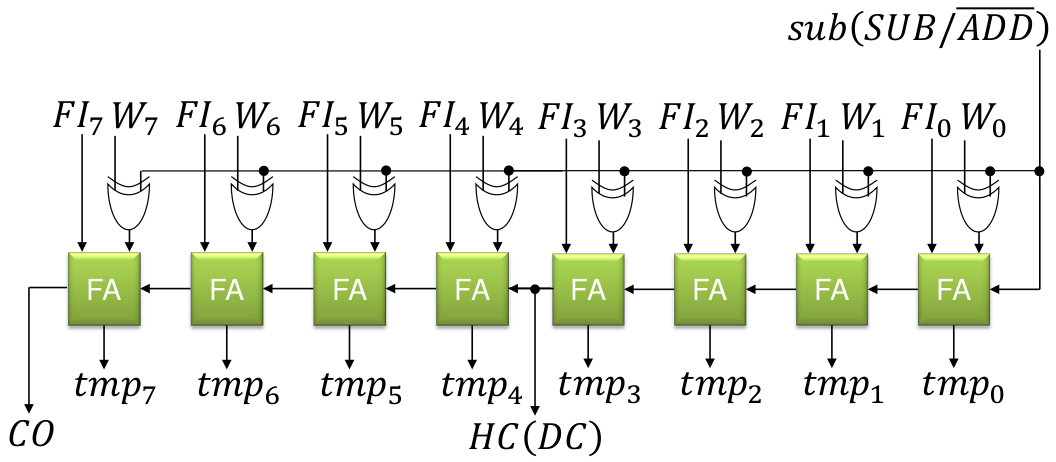Figure 3. ALU add sub 

Code 3. Up to add and sub

```always @( CB or FI or W or HC or CI or bit_mask or sub )
begin
HC = 1'b0;
casex( CB ) `
IPSW: tmp = { 1'b0, W }; // Pass W register value
`ICLR: tmp = 9'b0_0000_0000; // Clear
`IADD, `ISUB:
begin { HC, tmp[3:0] }= {1'b0,FI[3:0]} + {1'b0, sub? ~W[3:0]:W[3:0]} + sub;
tmp[8:4] = {1'b0,FI[7:4]} + {1'b0, sub? ~W[7:4]:W[7:4]} + HC;
end
```

The verilog design of the ALU is based on the diagram on Figure 2. The input, output, and process looks clear which was implemented on Code 1, however the detail operation within the bitmask, ALU, and w register should be examined on Code 2, Code 3, and Code 4. The ALU operates between the value on the W register and the current input FI. For addition and subtraction, Code 3 should follow the diagram on Figure 3, while for other operations are not as complicated which is on Code 4. After that, the output can be written on Code 5. The operation definitions are available on Code 6 which the bit opcode from 2nd to 6th from left to right is used.

Code 4. Other Operations

````IDEC1, `IDEC2: tmp = { 1'b0, FI } - 1 ; // Decrement, Decrement and skip if 0
`IOR : tmp = { 1'b0, FI } | { 1'b0, W } ; // Logical OR
`IAND: tmp = { 1'b0, FI } & { 1'b0, W } ; // Logical AND
`IXOR: tmp = { 1'b0, FI } ^ { 1'b0, W } ; // Logical Exclusive OR
`IPSF: tmp = { 1'b0, FI } ; // Pass FI
`INTF: tmp = { 1'b0, ~FI } ; // Complement FI
`IINC1, `IINC2: tmp = { 1'b0, FI } + 1 ; // Increment, Increment and skip if 0
`IRRF: tmp = {FI, CI, FI[7:1]} ; // Rotate Right through Carry
`IRLF: tmp = {FI, CI} ; // Rotate Left through Carry
`ISWP: tmp = {1'b0, FI[3:0], FI[7:4]}; // nibble swap
`IBCF: tmp = {1'b0, FI} & {1'b0,~bit_mask} ; // bit clear
`IBSF: tmp = {1'b0, FI} | {1'b0, bit_mask} ; // bit set
`IBTF: tmp = {1'b0, FI} & {1'b0, bit_mask} ; // bit test
default: tmp = 9'bx_xxxx_xxxx;
end case
end
```

Code 5. Output, W Register, and Flags

```// FO
assign FO = tmp[7:0] ;
// W Register
always @( posedge CLK )
if( WE ) W <= tmp[7:0] ; // Flag
assign CO = tmp ; // Carry Borrow flag
assign DC = HC ; // Half carry flag
assign Z = (tmp[7:0] == 0) ; // Zero flag
endmodule
```

Code 6. ALU Operation Definition

````define IPSW 5'b00000 // Pass W
`define ICLR 5'b00001 // Clear
`define ISUB 5'b00010 // Sub
`define IDEC1 5'b00011 // Dec
`define IOR 5'b00100 // Or
`define IAND 5'b00101 // And
`define IXOR 5'b00110 // Xor
`define IADD 5'b00111 // Add
`define IPSF 5'b01000 // Pass F
`define INTF 5'b01001 // Not
`define IINC1 5'b01010 // Inc
`define IDEC2 5'b01011 // Dec
`define IRRF 5'b01100 // Rotate Right with carry
`define IRLF 5'b01101 // Rotate Left with carry
`define ISWP 5'b01110 // Nibble swap
`define IINC2 5'b01111 // Inc
`define IBCF 5'b100?? // Bit Clear F
`define IBSF 5'b101?? // Bit Set F
`define IBTF 5'b11??? // Bit Test F
```

### 2.2 Core Input, Output, and Register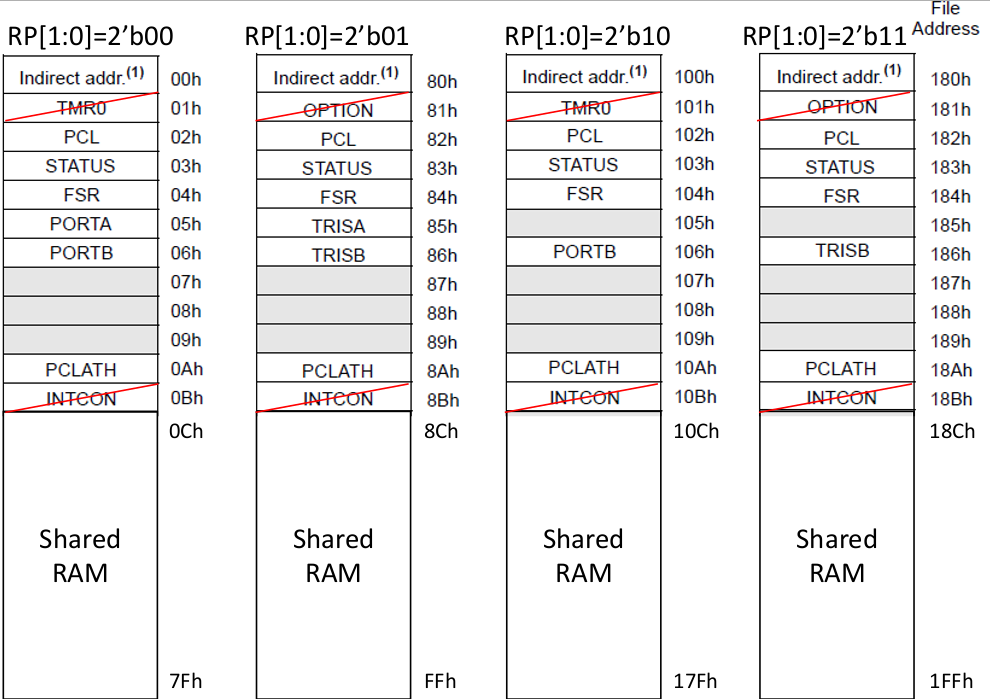Figure 4. Data Memory Map  

Code 7. Input, Output, and Register for Core Module

```// STATUS Register
`define STATUS { IRP, RP, nTO, nPD, Z, DC, C }
// IR
`define IRB IR[ 9:7]
`define IRK IR[ 7:0]
`define IRF IR[ 6:0]
`define IRA IR[10:0]
// Memory Address
`define A_INDF 7'b000_0000
`define A_PCL 7'b000_0010

module pic16core ( CLK, RST, RA, RB );
input CLK;
input RST;
inout [7:0] RA;
inout [7:0] RB;

parameter PROG = "program.mem";

// Special Register
reg IRP;
reg [1:0] RP;
reg nTO, nPD, Z, DC, C; // 03 83 // STATUS
reg [7:0] FSR; // 04 84
reg [7:0] PORTA, TRISA; // 05 85
reg [7:0] PORTB, TRISB; // 06 86
reg [4:0] PCLATH; // 0A 8A
reg [7:0] RAM[ 12 : 127 ]; // 0C-7F // DATA bus
reg [7:0] SDATA; // for special register
reg [7:0] RDATA; // for ALU operand
wire [7:0] WDATA; // for ALU result
wire [7:0] DDATA; // for Data RAM Read Data

// Flag data from ALU to Flag register
wire CO, DCO, ZO; // Control Signal
reg NOP_S; // Fetch cancel on CALL, GOTO
reg [4:0] ALU_CB; // ALU control
reg W_W; // Write enable for W register
reg C_W, DC_W, Z_W; // Write enable for Flag register (STATUS[2:0])
reg F_W; // Write enable for Data memory
reg WDT_C; // WDT clear
reg nTO_S, nTO_C; // nTO set and clear
reg nPD_S, nPD_C; // nPD set and clear
reg SLEEP; // Sleep mode
reg SLP_S; // Sleep mode set // Register
reg [12:0] PC; // Program Counter { PCH, PCL }
reg [13:0] IR; // Instruction Register

// Register
reg [12:0] PC; // Program Counter { PCH, PCL }
reg [13:0] IR; // Instruction Register
```

### 2.3 Effective Addressing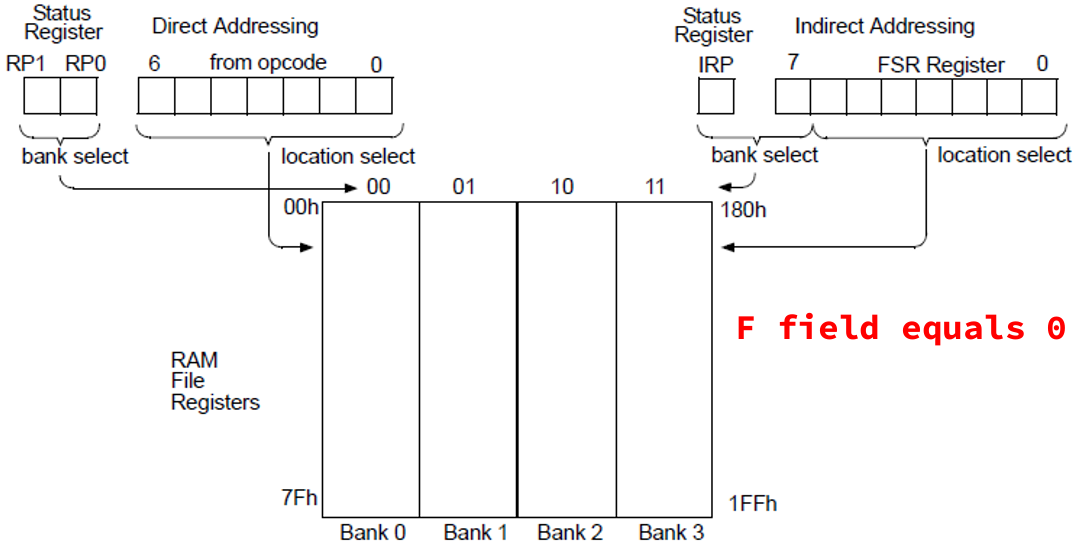Figure 5. Direct and indirect addressing diagram  

Based on Figure 5, Code 8 should write to RP if direct addressing, otherwise if indirect addressing IRP should be FSR.

Code 8. Affective addressing for core module

```// Effective Address
wire [ 8 : 0 ] EA;
assign EA = ( `IRF == 0 ) ? { IRP , FSR [7:0] } : { RP , `IRF};
```

### 2.4 Program Counter and Return Stack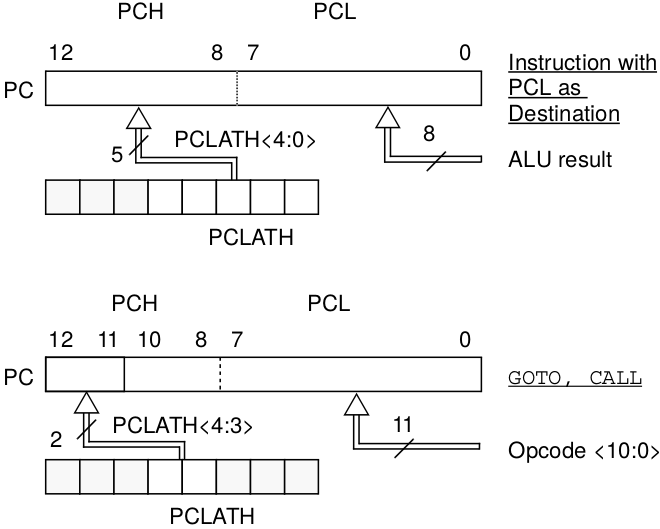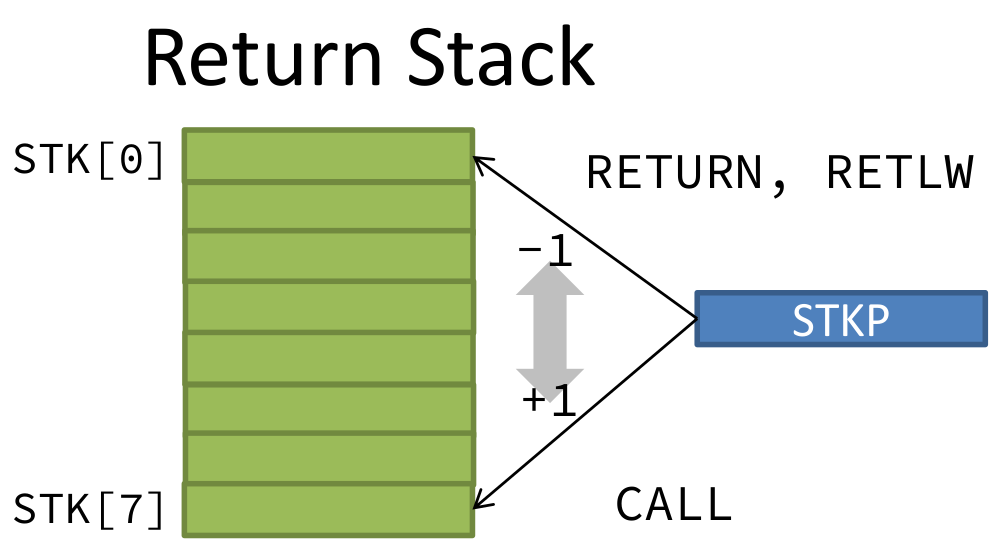Figure 6. PC loading and return stack  

For Code 9 about program counter and return stack, the value of PC is based the left diagram of Figure 6. When operation call, then the stack is pushed, and when operation return, then the stack is popped. The value of STKP should be based on the right diagram of Figure 6.

Code 9. Program counter and return stack

```// Program Counter & Return Stack
reg [ 12:8 ] STK[0:7]; // Return Stack depth 8
reg [ 2:0] STKP; // Return stack pointer 4 bit
reg STK_PU; // Stack Push enable
reg STK_PO; // Stack Pop enable
reg PC_W; // Write enable for CALL, GOTO

// Program Counter
always @( posedge CLK )
if( RST ) PC <= 0; else // RESET
if( PC_W ) PC <= {PCLATH[4:3],IR[10:0] }; else // CALL, GOTO
if( F_W && EA [6:0] == `A_PCL ) PC <= { PCLATH[4:3], WDATA[7:0] }; else // write PCL register if( STK_PO ) PC <= STK[ STKP-1 ]; else // RETURN, RETLW
if( SLEEP || SLP_S ) PC <= PC ; else // SLEEP mode
PC <= PC + 1;

// Return Stack
always @( posedge CLK )
begin
if( RST ) STKP<= 0 ; else // for Empty
if( STK_PU ) begin STK[ STKP ] <= PC ; STKP<= STKP+1; end else // for CALL
if( STK_PO ) STKP<= STKP-1; // for RETxx
end
```

### 2.5 Instruction Memory and Register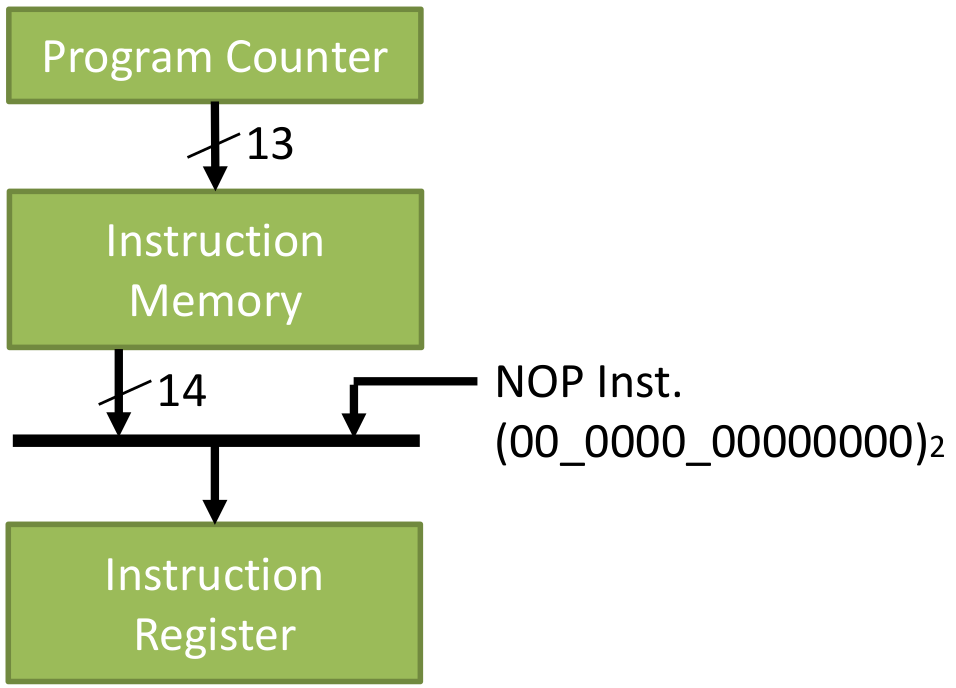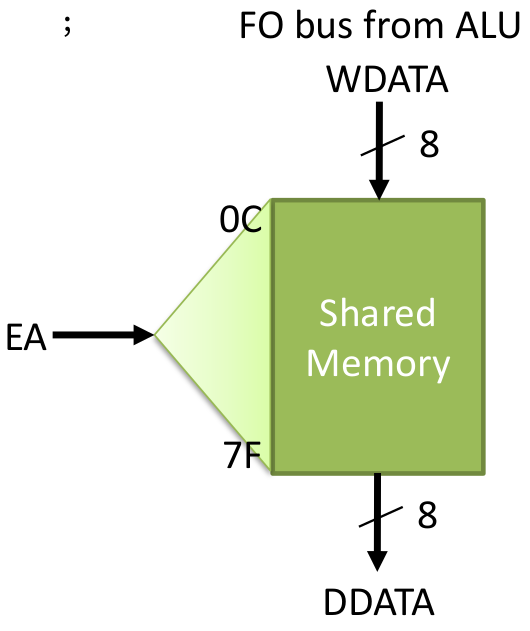Figure 7. Left image is about instruction register and right image is about shared memory 

Code 10. Instruction memory and Register

```// Instruction Memory (8k word)
reg [ 13 : 0 ] IMEM[ 0 : 8195 ];
initial
begin
\$readmemh( PROG, IMEM );
end

// Instruction Register
always @( posedge CLK )
if( NOP_S || PC_W || STK_PO || RST )
IR <= 14'b00_0000_0000_0000 ; else // if CALL, RET, cond.SKIP
IR <= IMEM[PC] ; // Instruction fetch
```

### 2.6 Decode and Control

To write Code 11, the instruction table and instruction details on the datasheet  should be referred. Code 11 is written starting from first two bits of the instructions then the next 4 bits of the instructions. Refer again to the datasheet  of which status are affected. Unfortunately, sleep here is a repeat NOP.

Code 11. Decode and control

```// Decode & Control
always @( IR or ZO )
begin
ALU_CB=IR[ 12 : 8 ];
F_W=0; W_W=0; Z_W=0; DC_W=0; C_W=0; nTO_S=0; nTO_C=0; nPD_S=0; nPD_C=0; STK_PU=0;
STK_PO=0; NOP_S=0; PC_W=0; WDT_C=0; SLP_S=0;
case( IR[ 13 : 12] )
2'b00 :
begin
W_W = ~IR && IR[11:8] != 4'b0000 ;
F_W = IR; //same with W_W = IR && |IR[11:8] meaning bit 8 is 1 and bit 9-12 isn`t 0
case( IR[ 11 : 8 ] )
4'b0000:
case( IR )
1'b0: case( IR[ 6 : 0 ] )
7'b000_1000 : begin NOP_S=1 ; STK_PO=1 ; end // RETURN
// 7'b000_1001: ; // RETFIE
7'b110_0011 : begin nTO_S=1; nPD_S=1; WDT_C=1; SLP_S=1 ; NOP_S=1 ; end // SLEEP
// 7'b110_0100: ; // CLRWDT
default: ; // NOP
endcase
1'b1: ; // MOVWF f
endcase
4'b0001: begin Z_W=1 ; end // CLRW, CLRF
4'b0010: begin C_W=1 ; DC_W=1 ; Z_W=1 ; end // SUBWF
4'b0011: begin Z_W=1 ; end // DECF
4'b0100: begin Z_W=1 ; end // IORWF
4'b0101: begin Z_W=1 ; end // ANDWF
4'b0110: begin Z_W=1 ; end // XORWF
4'b0111: begin C_W=1 ; DC_W=1 ; Z_W=1 ; end // ADDWF
4'b1000: begin Z_W=1 ; end // MOVF
4'b1001: begin Z_W=1 ; end // COMF
4'b1010: begin Z_W=1 ; end // INCF
4'b1011: begin NOP_S= (ZO==1) ? 1 : 0 ; end // DECFSZ
4'b1100: begin C_W=1 ; end // RRF
4'b1101: begin C_W=1 ; end // RLF
4'b1110: ; // SWPF
4'b1111: begin NOP_S=ZO ; end // INCFSZ
endcase //IR
end
2'b01 :
begin
case( IR[ 11 : 10 ] )
2'b00 : F_W = 1 ; // BCF f, b
2'b01 : F_W = 1 ; // BSF f, b
2'b10 : NOP_S=ZO ; // BTFSC f, b
2'b11 : NOP_S=~ZO ; // BTFSS f, b
endcase
end
2'b10 :
begin
PC_W = 1 ; NOP_S = 1 ;
case( IR[ 11 ] )
1'b0 : STK_PU = 1 ; // CALL
1'b1 : ; // GOTO
endcase
end
2'b11 :
begin
W_W= 1 ;
casex( IR[ 11 : 8 ] )
4'b00xx : begin ALU_CB=`IPSF ; end // MOVLW k //--> pass in ALU to W //
4'b01xx : begin ALU_CB=`IPSF ; STK_PO = 1 ; NOP_S = 1; end // RETLW k
4'b1000 : begin ALU_CB=`IOR ; Z_W = 1 ; end // IORLW k
4'b1001 : begin ALU_CB=`IAND ; Z_W = 1 ; end // ANDLW k
4'b1010 : begin ALU_CB=`IXOR ; Z_W = 1 ; end // XORLW k
4'b110x : begin ALU_CB=`ISUB ; C_W=1 ; DC_W=1 ; Z_W = 1 ; end // SUBLW k
4'b111x : begin ALU_CB=`IADD ; C_W=1 ; DC_W=1 ; Z_W = 1 ; end // ADDLW k
endcase
end
endcase
end // always @ ( IR or ZO )
```

### 2.7 Special Register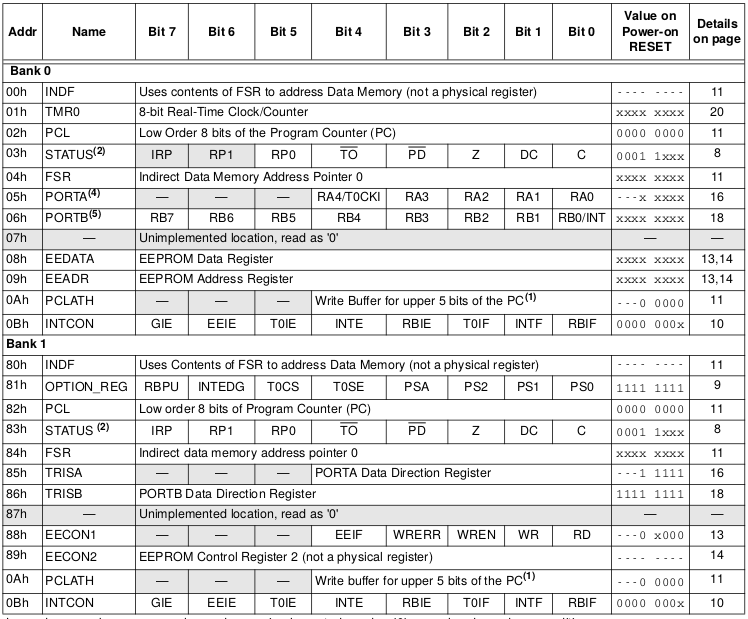Figure 8. Special register 

Code 12 about special register is based on Figure 3 memory map for the written bits and Figure 8 about special register itself for its values.

Code 12. Special register

```// Special Register
// Write
always @( posedge CLK or posedge RST )
begin
if( RST )
begin
C = 0 ;
DC = 0 ;
Z = 0 ;
IRP = 0 ;
RP = 2'b00 ;
nTO = 1 ; // nTO=1 on Power-on
nPD = 1 ; // nPD=1 on Power-on
FSR = 0 ; PCLATH = 5'b00000 ;
PORTA = 8'b0000_0000 ;
TRISA = 8'b1111_1111 ; // All ports for input
PORTB = 8'b0000_0000 ;
TRISB = 8'b1111_1111 ; // Table page 18-20
// All ports for input
end
else
begin
// STATUS
if( C_W ) C = CO ;
if( DC_W ) DC = DCO ;
if( Z_W ) Z = ZO ;
if( nPD_S ) nPD = 1'b1;
if( nPD_C ) nPD = 1'b0;
if( nTO_S ) nTO = 1'b1;
if( nTO_C ) nTO = 1'b0;
// Register Write
if( F_W ) //pic16_behaviour page 41
casex( EA ) //effective address
// 9'b?0_000_0001: TMR0 = WDATA; // 01 101 Described TMR0 part //find in address table
// 9'b?1_000_0001:`OPTION = WDATA; // 81 181
// 9'b??_000_0010: PCL = WDATA; // 02 82 102 182 Described PC part
9'b??_000_0011:`STATUS = WDATA; // 03 83 103 183
9'b??_000_0100: FSR = WDATA; // 04 84 104 184
9'b00_000_0101: PORTA = WDATA; // 05
9'b01_000_0101: TRISA = WDATA; // 85
9'b?0_000_0110: PORTB = WDATA; // 06 106
9'b?1_000_0110: TRISB = WDATA; // 86 186
// 9'b??_000_0111: ; // 07
// 9'b??_000_1000: ; // 08 EEDATA not implement
// 9'b??_000_1001: ; // 09 EEADR not implement
9'b??_000_1010: PCLATH = WDATA[4:0]; // 0A 8A 10A 18A
// 9'b??_000_1011:`INTCON = WDATA; // 0B 8B 10B 18B
endcase
end
end
```

Code 13. Data RAM

```// Data RAM (Write)
always @( posedge CLK )
begin
if( F_W && ( EA[6:0] >= 7'b001_1000 ) ) RAM[ EA[6:0] ] <= WDATA ; //12-127 PIC16 behaviour p.46 //light behaviour p.41 //Effective Address only 7bit not 9bit start from 12 //store RAM to WDATA
end
// Selecter for Special Register
always @( IR or PC or EA or IRP or RP or nTO or nPD or Z or DC or C // STATUS
or FSR or RA or TRISA or RB or TRISB or PCLATH )
casex( EA )
// 9'b?0_000_0001: SDATA = TMR0;
// 9'b?1_000_0001: SDATA =`OPTION;
9'b??_000_0010: SDATA = PC[7:0] ; // PCL
9'b??_000_0011: SDATA =`STATUS ; // STATUS
9'b??_000_0100: SDATA = FSR ; // FSR
9'b00_000_0101: SDATA = PORTA ; // RA
9'b01_000_0101: SDATA = TRISA ; // TRISA
9'b?0_000_0110: SDATA = PORTB ; // RB
9'b?1_000_0110: SDATA = TRISB ; // TRISB
9'b??_000_1010: SDATA = {3'b000, PCLATH }; // PCLATH //because SDATA is 9 bit and PCLATH is only 6 bit, we need to add 3 bit before PCLATH // 9'b??_000_1011: SDATA =`INTCON;
default: SDATA = 8'bxxxx_xxxx;
endcase
// Data RAM Read
assign DDATA = RAM[EA[7:0]] ;
```

### 2.8 Data Path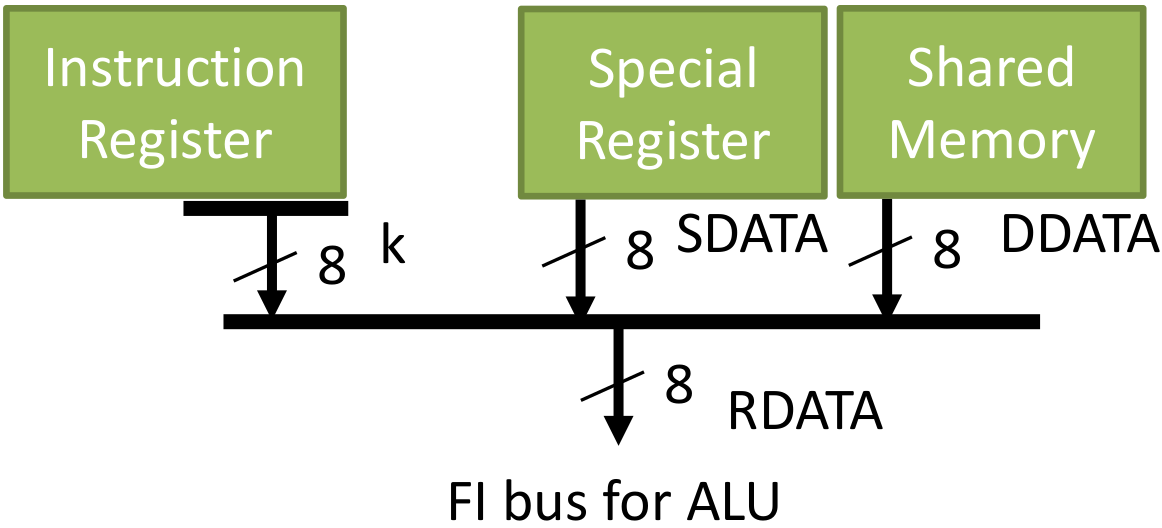Figure 9. Data path to ALU 

Code 14. Data selector for ALU

```// Data selector for ALU operand
always @( IR or EA or DDATA or SDATA )
begin RDATA <= 8'bxxxx_xxxx ;
if( &IR[13:12] ) RDATA <= `IRK ; else //
casex( EA )
9'b??_000_0010: RDATA <= SDATA ; // PCL
9'b??_000_0011: RDATA <= SDATA ; // STATUS
9'b??_000_0100: RDATA <= SDATA ; // FSR
9'b0?_000_0101: RDATA <= SDATA ; // PORTA, TRISA
9'b0?_000_0110: RDATA <= SDATA ; // PORTB, TRISB
9'b??_000_1010: RDATA <= SDATA ; // PCLATH
default: RDATA <= RAM[EA[7:0]] ; // Shared memory
endcase
end
```

### 2.9 ALU Initiate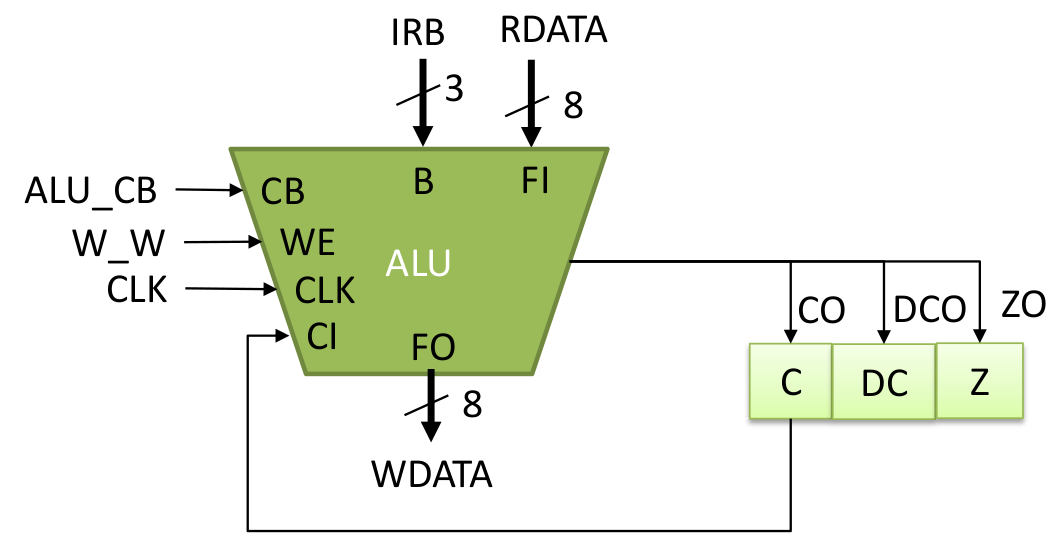Figure 10. ALU initiate diagram 

Code 15. ALU initiate code

```// Execute
alu i_alu ( .CLK(CLK), .CB(ALU_CB), .WE(W_W), .B(`IRB),
.FI(RDATA),
.FO(WDATA),
.CI(C), .CO(CO), .DC(DCO), .Z(ZO) );
```

### 2.10 Sleep

Back on Code 11, sleep is a repeat NOP. Here on Figure 9, waking up from sleep is not implemented, sleep forever but can be reset.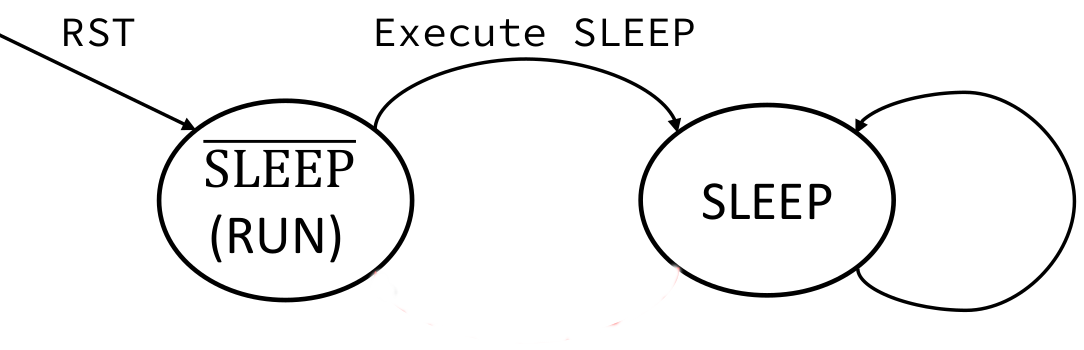Figure 11. Sleep diagram 

Code 16. Sleep implementation

```// Sleep mode
always @( posedge CLK or posedge RST )
begin
if(RST) SLEEP <= 0; else //0 mean run
if(SLP_S) SLEEP <= 1; //1 mean execute the sleep mode
end
```

### 2.11 Tristate Buffer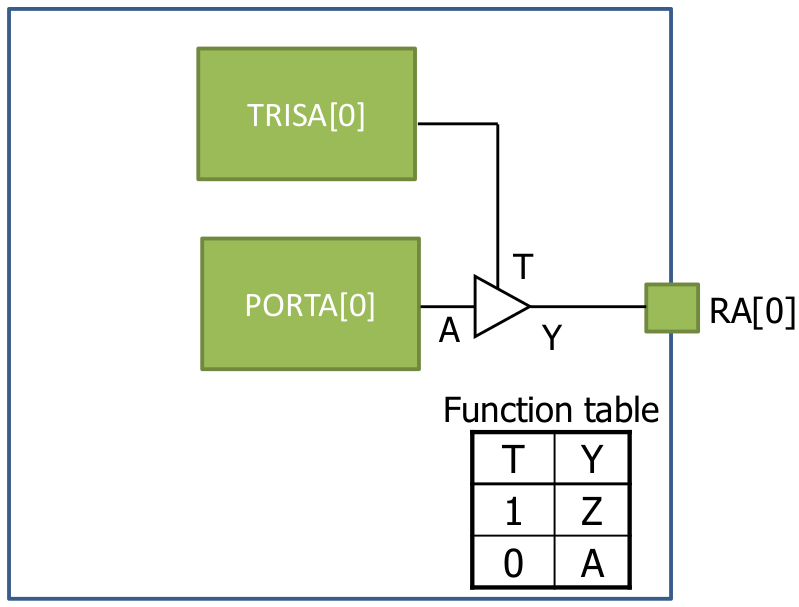Figure 12. Tristate buffer diagram 

Code 17. Tristate buffer implementation

```// Tristate buffer for GPIO
assign RA = ( TRISA ) ? 1'bZ : PORTA;
assign RA = ( TRISA ) ? 1'bZ : PORTA;
assign RA = ( TRISA ) ? 1'bZ : PORTA;
assign RA = ( TRISA ) ? 1'bZ : PORTA;
assign RA = ( TRISA ) ? 1'bZ : PORTA;
assign RA = ( TRISA ) ? 1'bZ : PORTA;
assign RA = ( TRISA ) ? 1'bZ : PORTA;
assign RA = ( TRISA ) ? 1'bZ : PORTA;
assign RB = ( TRISB ) ? 1'bZ : PORTB;
assign RB = ( TRISB ) ? 1'bZ : PORTB;
assign RB = ( TRISB ) ? 1'bZ : PORTB;
assign RB = ( TRISB ) ? 1'bZ : PORTB;
assign RB = ( TRISB ) ? 1'bZ : PORTB;
assign RB = ( TRISB ) ? 1'bZ : PORTB;
assign RB = ( TRISB ) ? 1'bZ : PORTB;
assign RB = ( TRISB ) ? 1'bZ : PORTB;
endmodule
```

## 3. Simulation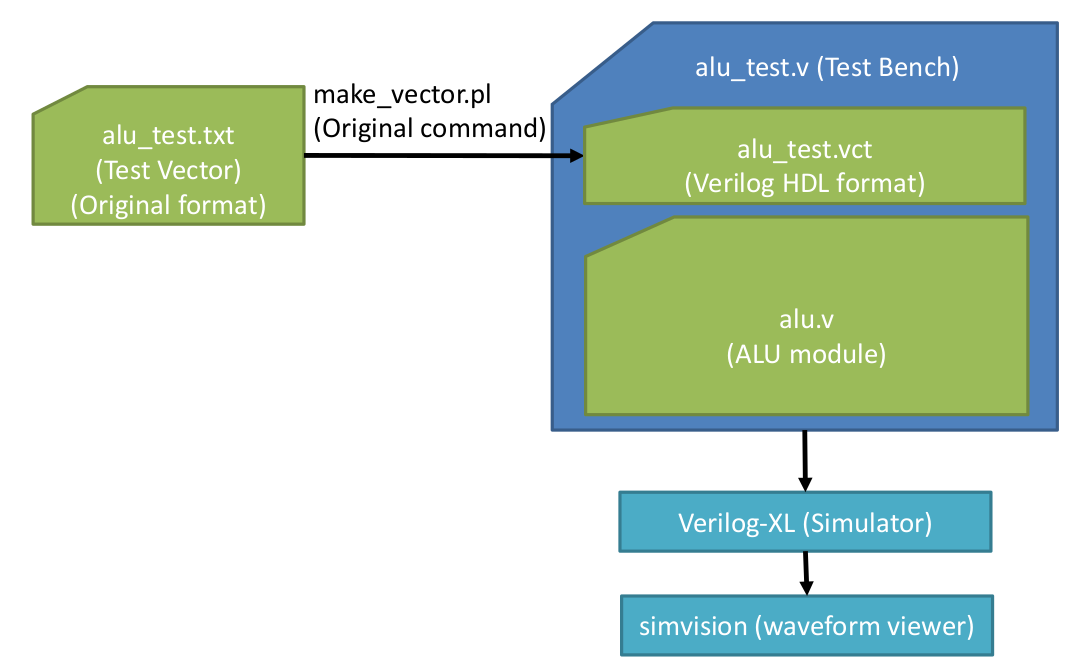Figure 13. Test simulation of the ALU code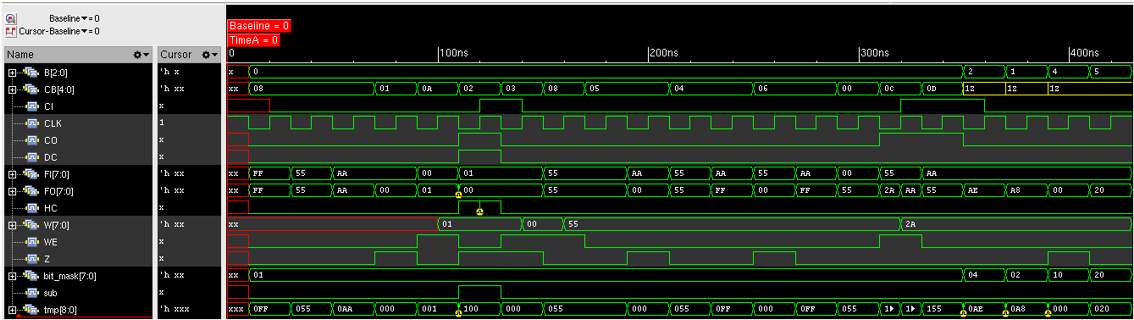Figure 14. Waveform in simvision for ALU 

All the codes to conduct the simulation are available online . For solely testing the ALU, follow Figure 11 which are about generating the clock, and testing operations starting from PASSF, subtraction, until bit test. Figure 13 compiles the test sequence from text format into verilog HDL format using make_vector.pl binary. Then these files including Code 1-6 is compiled using verilog binary. The waves can be examined using simvision which can be shown on Figure 14. All the wave values are shown in hexadecimals. CB shows the executed operation. It is seen the W register becomes 1 when performed an increment operation, and reduced to 0 when subtract operation was performed, note that HC and CO has started to become affected. After that is logical operation where the result can be seen on FO as well. In the ends of this simulation is where the bit manipulation operations are performed where the B and bitmask variables are affected.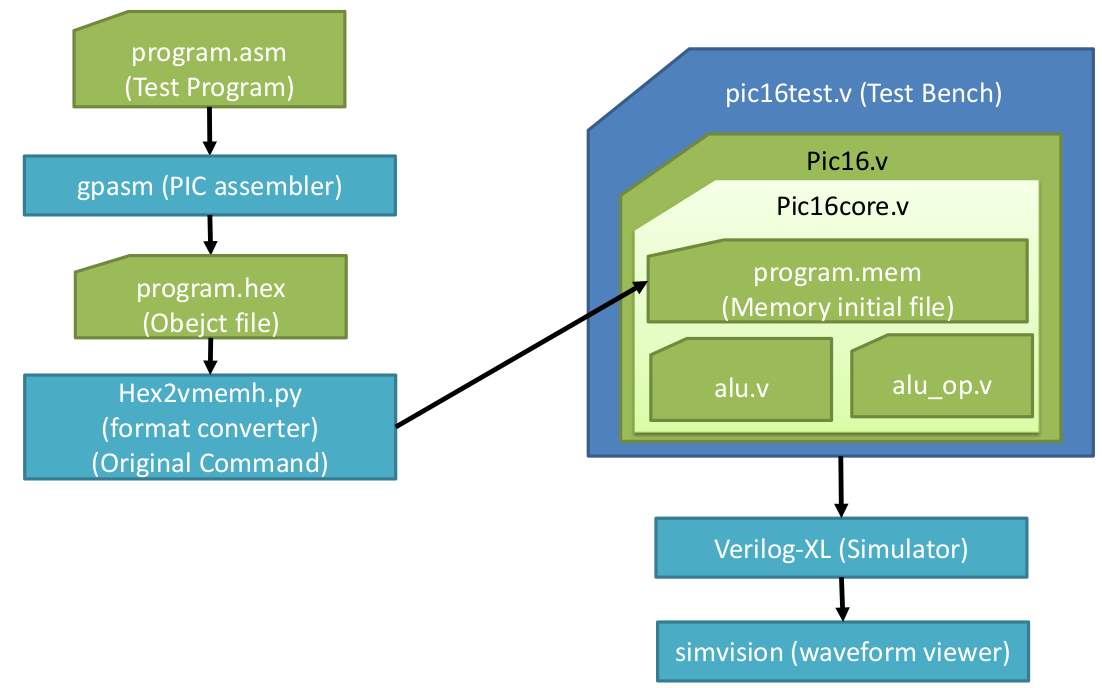Figure 15. Test simulation of PIC core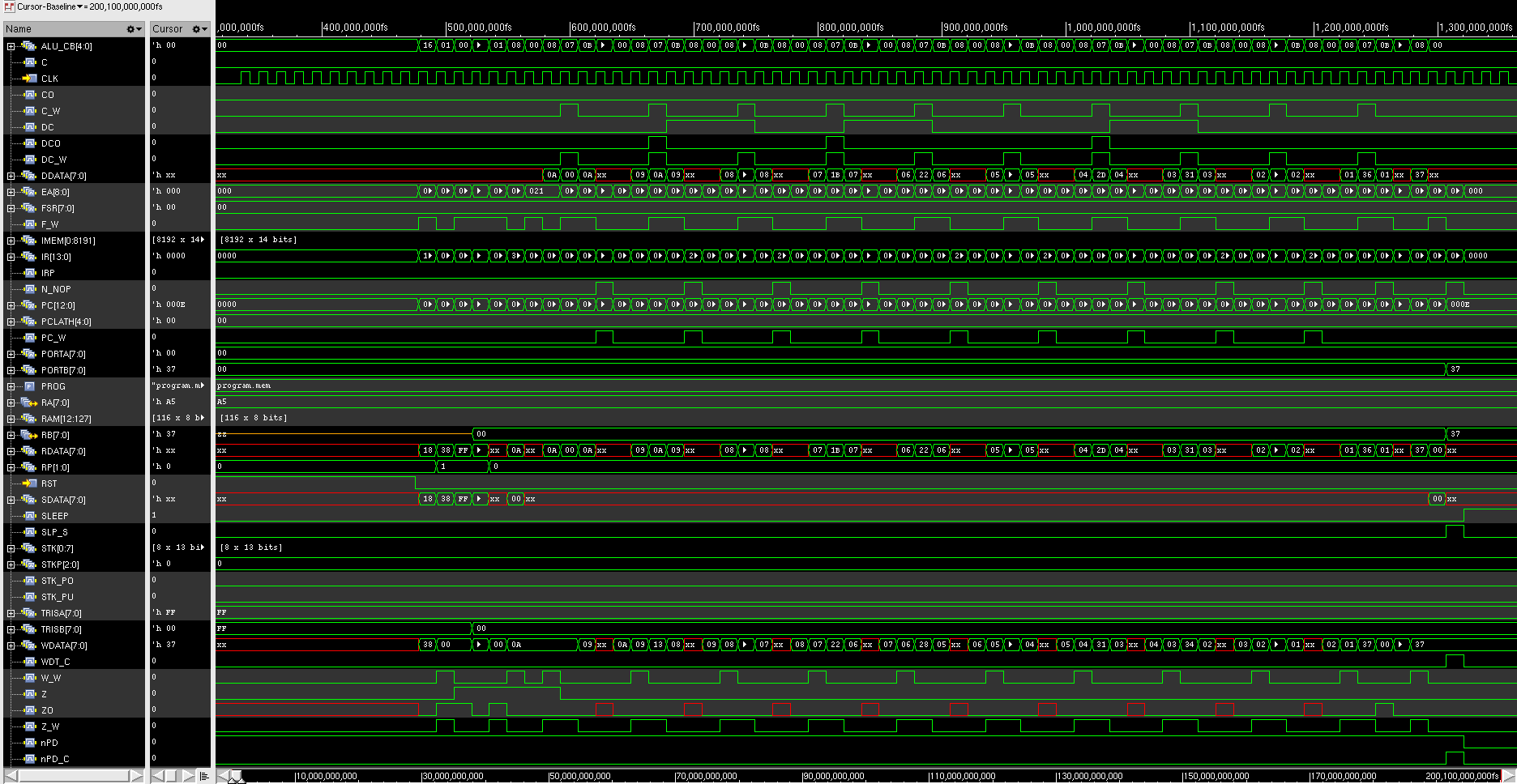Figure 16. Waveform in simvision for PIC core 

Figure 15 shows the diagram of testing the PIC16 core. The program.asm shows that only 10 operations are tested . Next it have to be converted into an assembly file using gpasm which then the format have to be converted. After that the PIC16 core whole files  can be compiled using verilog and the waves can be seen using simvision on Figure 16. The first part of the test should bitset the RP, clear W, set TRISB to 00h, bitclear RP. The next operations are to do ten times addition of ten. DData, RData, and WData should look consistent. First the value should be 0A which is hexadecimal of 10, then it should increase to 1B and everytime added by 10. Note that the decrements are also shown from 0A until 01. In the end the result is 37 and will be transferred to PORTB. The last operation is sleep. Note that the design on this report does not implement everything from the original as shown on Table 1.

Table 1. Original PIC16 versus this report’s design

PIC16Original ThisReport’s Design
Pipeline 4 cycles /1 stage 1 cycle / 1stage
Clock Selectable Externalonly
Sleep mode Low powermode Repeat NOP
Watch DogTimer Available None
Timer Available None
Prescaler Available None
Interrupt Available None
Flashmemory Available None

## 4. Implementation

The verilog HDL codes can be implemented in FPGA. On this report Nexys4 DDR board is used on Figure 15 and Vivado software is used to synthesize the code. The LED should show 110111(2) which is 37 that is the result of the addition.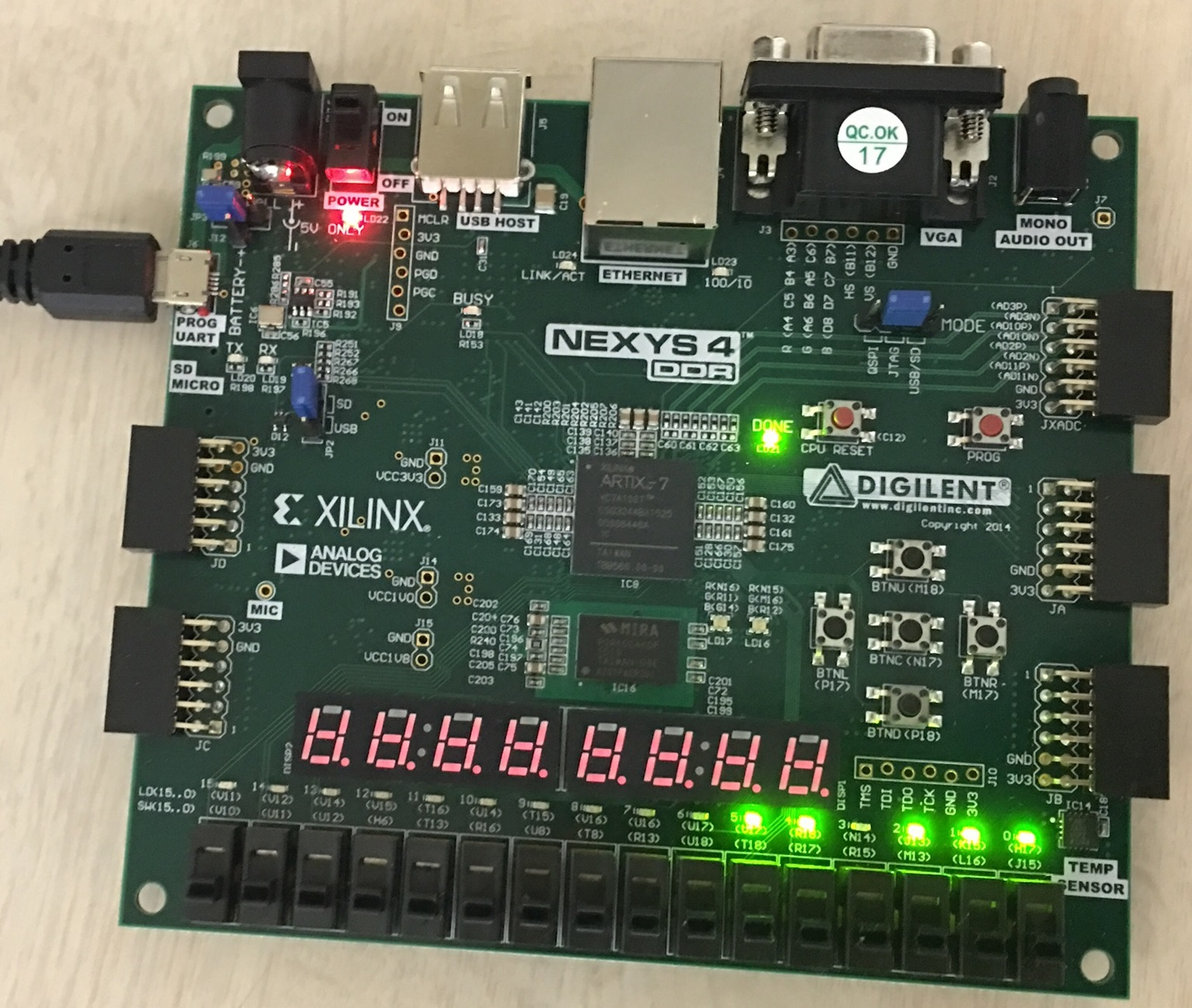Figure 17. Nexys4 DDR board

## 5. Reference

1. https://en.wikipedia.org/wiki/PIC_microcontroller
2. M. Kuga, “PIC_behavior20180124”, Supplied Course Material, 24-01-2018.
3. Microchip, “16F84A-35007b”, PIC16F84A Data Sheet , 2001.
4. https://github.com/0fajarpurnama0/Programming-Practice/tree/master/verilog/PIC16